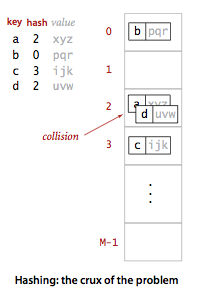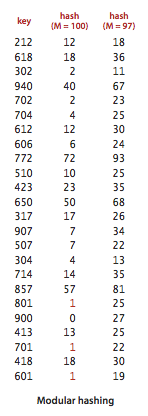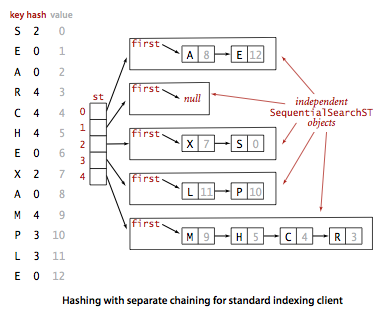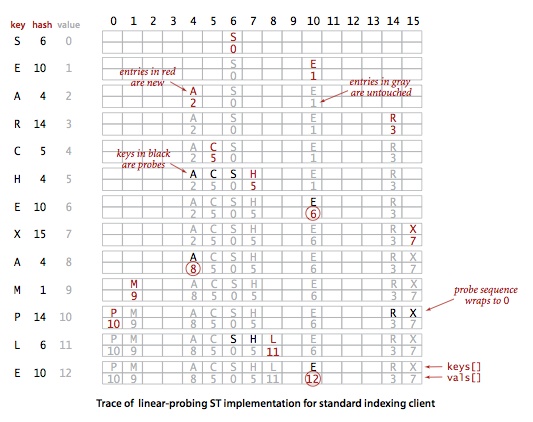# 3.4   Hash Tables

If keys are small integers, we can use an array to implement a symbol table, by interpreting the key as an array index so that we can store the value associated with key i in array position i. In this section, we consider hashing, an extension of this simple method that handles more complicated types of keys. We reference key-value pairs using arrays by doing arithmetic operations to transform keys into array indices.Search algorithms that use hashing consist of two separate parts. The first step is to compute a hash function that transforms the search key into an array index. Ideally, different keys would map to different indices. This ideal is generally beyond our reach, so we have to face the possibility that two or more different keys may hash to the same array index. Thus, the second part of a hashing search is a collision-resolution process that deals with this situation.## Hash functions.

If we have an array that can hold M key-value pairs, then we need a function that can transform any given key into an index into that array: an integer in the range [0, M-1]. We seek a hash function that is both easy to compute and uniformly distributes the keys.
• Typical example. Suppose that we have an application where the keys are U.S. social security numbers. A social security number such as 123-45-6789 is a 9-digit number divided into three fields. The first field identifies the geographical area where the number was issued (for example number whose first field are 035 are from Rhode Island and numbers whose first field are 214 are from Maryland) and the other two fields identify the individual. There are a billion different social security numbers, but suppose that our application will need to process just a few hundred keys, so that we could use a hash table of size M = 1000. One possible approach to implementing a hash function is to use three digits from the key. Using three digits from the field on the right is likely to be preferable to using the three digits in the field on the left (since customers may not be equally dispersed over geographic areas), but a better approach is to use all nine digits to make an int value, then consider hash functions for integers, described next.

• Positive integers. The most commonly used method for hashing integers is called modular hashing: we choose the array size M to be prime, and, for any positive integer key k, compute the remainder when dividing k by M. This function is very easy to compute (k % M, in Java), and is effective in dispersing the keys evenly between 0 and M-1.

• Floating-point numbers. If the keys are real numbers between 0 and 1, we might just multiply by M and round off to the nearest integer to get an index between 0 and M-1. Although it is intuitive, this approach is defective because it gives more weight to the most significant bits of the keys; the least significant bits play no role. One way to address this situation is to use modular hashing on the binary representation of the key (this is what Java does).

• Strings. Modular hashing works for long keys such as strings, too: we simply treat them as huge integers. For example, the code below computes a modular hash function for a String s, where R is a small prime integer (Java uses 31).
 int hash = 0; for (int i = 0; i < s.length(); i++) hash = (R * hash + s.charAt(i)) % M; 

• Compound keys. If the key type has multiple integer fields, we can typically mix them together in the way just described for String values. For example, suppose that search keys are of type USPhoneNumber.java, which has three integer fields area (3-digit area code), exch (3-digit exchange), and ext (4-digit extension). In this case, we can compute the number
 int hash = (((area * R + exch) % M) * R + ext) % M; 

• Java conventions. Java helps us address the basic problem that every type of data needs a hash function by requiring that every data type must implement a method called hashCode() (which returns a 32-bit integer). The implementation of hashCode() for an object must be consistent with equals. That is, if a.equals(b) is true, then a.hashCode() must have the same numerical value as b.hashCode(). If the hashCode() values are the same, the objects may or may not be equal, and we must use equals() to decide which condition holds.

• Converting a hashCode() to an array index. Since our goal is an array index, not a 32-bit integer, we combine hashCode() with modular hashing in our implementations to produce integers between 0 and M-1 as follows:
 private int hash(Key key) { return (key.hashCode() & 0x7fffffff) % M; } 
The code masks off the sign bit (to turn the 32-bit integer into a 31-bit nonnegative integer) and then computing the remainder when dividing by M, as in modular hashing.

• User-defined hashCode(). Client code expects that hashCode() disperses the keys uniformly among the possible 32-bit result values. That is, for any object x, you can write x.hashCode() and, in principle, expect to get any one of the 2^32 possible 32-bit values with equal likelihood. Java provides hashCode() implementations that aspire to this functionality for many common types (including String, Integer, Double, Date, and URL), but for your own type, you have to try to do it on your own. Program PhoneNumber.java illustrates one way to proceed: make integers from the instance variables and use modular hashing. Program Transaction.java illustrates an even simpler approach: use the hashCode() method for the instance variables to convert each to a 32-bit int value and then do the arithmetic.
We have three primary requirements in implementing a good hash function for a given data type:

• It should be deterministic—equal keys must produce the same hash value.

• It should be efficient to compute.

• It should uniformly distribute the keys.
To analyze our hashing algorithms and develop hypotheses about their performance, we make the following idealized assumption.

## Assumption J (uniform hashing assumption).

The hash function that we use uniformly distributes keys among the integer values between 0 and M-1.

## Hashing with separate chaining.

A hash function converts keys into array indices. The second component of a hashing algorithm is collision resolution: a strategy for handling the case when two or more keys to be inserted hash to the same index. A straightforward approach to collision resolution is to build, for each of the M array indices, a linked list of the key-value pairs whose keys hash to that index. The basic idea is to choose M to be sufficiently large that the lists are sufficiently short to enable efficient search through a two-step process: hash to find the list that could contain the key, then sequentially search through that list for the key.Program SeparateChainingHashST.java implements a symbol table with a separate-chaining hash table. It maintains an array of SequentialSearchST objects and implements get() and put() by computing a hash function to choose which SequentialSearchST can contain the key and then using get() and put() from SequentialSearchST to complete either job. Program SeparateChainingLiteHashST.java is similar but does it using an explict Node nested class.

Proposition K. In a separate-chaining hash table with M lists and N keys, the probability (under Assumption J) that the number of keys in a list is within a small constant factor of N/M is extremely close to 1. of N/M is extremely close to 1. (Assumes an idealistic hash function.)

This classical mathematical result is compelling, but it completely depends on Assumption J. Still, in practice, the same behavior occurs.

Property L. In a separate-chaining hash table with M lists and N keys, the number of compares (equality tests) for search and insert is proportional to N/M.

## Hashing with linear probing.

Another approach to implementing hashing is to store N key-value pairs in a hash table of size M > N, relying on empty entries in the table to help with with collision resolution. Such methods are called open-addressing hashing methods. The simplest open-addressing method is called linear probing: when there is a collision (when we hash to a table index that is already occupied with a key different from the search key), then we just check the next entry in the table (by incrementing the index). There are three possible outcomes:
• key equal to search key: search hit
• empty position (null key at indexed position): search miss
• key not equal to search key: try next entryProgram LinearProbingHashST.java is an implementation of the symbol-table ADT using this method.

As with separate chaining, the performance of open-addressing methods is dependent on the ratio α = N/M, but we interpret it differently. For separate chaining α is the average number of items per list and is generally larger than 1. For open addressing, α is the percentage of table positions that are occupied; it must be less than 1. We refer to α as the load factor of the hash table.

Proposition M. In a linear-probing has table of size M with N = α M keys, the average number of probes (under Assumption J) is ~ 1/2 (1 + 1 / (1 - α)) for search hits and ~ 1/2 (1 + 1 / (1 - α)^2) for search misses or inserts.

#### Q + A.

1. Why does Java use 31 in the hashCode() for String?
2. It's prime, so that when the user mods out by another number, they have no common factors (unless it's a multiple of 31). 31 is also a Mersenne prime (like 127 or 8191) which is a prime number that is one less than a power of 2. This means that the mod can be done with one shift and one subtract if the machine's multiply instruction is slow.
3. How do you extract the bits from a variable of type double for use in hashing?
4. Double.doubleToLongBits(x) returns a 64-bit long integer whose bit representation is the same as the floating-point representation of the double value x.
5. What's wrong with using (s.hashCode() % M) or Math.abs(s.hashCode()) % M to hash to a value between 0 and M-1?
6. The % operator returns a non-positive integer if its first argument is negative, and this would create an array index out-of-bounds error. Surprisingly, the absolute value function can even return a negative integer. This happens if its argument is Integer.MIN_VALUE because the resulting positive integer cannot be represented using a 32-bit two's complement integer. This kind of bug would be excruciatingly difficult to track down because it would only occur one time in 4 billion! [ The String hash code of "polygenelubricants" is -2^31. ]

#### Exercises

1. Is the following implementation of hashCode() legal?
 public int hashCode() { return 17; } 

Solution. Yes, but it would cause all keys to hash to the same spot, which would lead to poor performance.

2. Analyze the space usage of separate chaining, linear probing, and BSTs for double keys. Present your results in a table like the one on page 476.

Solution.

• Sequential search. 24 + 48N. A Node in a SequentialSearch symbol table consumes 48 bytes of memory (16 bytes overhead, 8 bytes key, 8 bytes val, 8 bytes next, and 8 bytes inner class overhead). A SequentialSearch object consumes 24 bytes (16 bytes overhead, 8 bytes first) plus the memory for the nodes.

Note that the booksite versions uses an extra 8 bytes per SequentialSearch object (4 for N and 4 for padding).

• Separate chaining. 56 + 32M + 48N. A SeparateChaining symbol table consumes 8M + 56 bytes (16 bytes overhead, 20 bytes array overhead, 8 bytes for pointer to array, 8 bytes per reference to each array entry, 4 bytes for M, 4 bytes for N, 4 bytes for padding), plus the memory for the M SequentialSearch objects.

#### Creative Exercises

1. Hash attack. Find 2^N strings, each of length N, that have the same hashCode() value, supposing the hashCode() implementation for String (as specified in the Java standard) is the following:
 public int hashCode() { int hash = 0; for (int i = 0; i < length(); i++) hash = (hash * 31) + charAt(i); return hash; } 

Solution. It is easy to verify that "Aa" and "BB" hash to the same hashCode() value (2112). Now, any string of length 2N that is formed by concatenating these two strings together in any order (e.g., BBBBBB, AaAaAa, BBAaBB, AaBBBB) will hash to the same value. Here is a list of 10000 strings with the same hash value.

2. Bad hash function. Consider the following hashCode() implementation for String, which was used in early versions of Java:
 public int hashCode() { int hash = 0; int skip = Math.max(1, length() / 8); for (int i = 0; i < length(); i += skip) hash = (hash * 37) + charAt(i); return hash; } 
Explain why you think the designers chose this implementation and then why you think it was abandoned in favor of the one in the previous exercise.

Solution. This was done in the hopes of computing the hash function more quickly. Indeed, the hash values were computed more quickly, but it became more likely that many strings hashed to the same values. This resulted in a significant degradation in performance on many real-world inputs (e.g., long URLs) which all hashed to the same value, e.g., http://www.cs.princeton.edu/algs4/34hash/*****java.html.

#### Web Exercises

1. Suppose we wish to repeatedly search a linked list of length N elements, each of which contains a very long string key. How might we take advantage of the hash value when searching the list for an element with a given key? Solution: precompute the hash value of each string in the list. When searching for a key t, compare its hash value to the hash value of a string s. Only compare the string s and t if their hash values are equal.
2. Implement hashCode() and equals() for the following data type. Be careful since it is likely that many of the points will have small integers for x, y, and z.
 public class Point2D { private final int x, y; ... } 
Answer: one solution would to make the first 16 bits of the hash code be the xor of the first 16 bits of x and the last 16 bits of y, and make the last 16 bits of the hash code be the xor of the last 16 bits of x and the first 16 bits of y. Thus, if x and y are only 16 bits or less, the hashCode values will be different for different points.
3. What is wrong with the following implementation of equals() for points?
 public boolean equals(Point q) { return x == q.x && y == q.y; } 
Wrong signature for equals(). It is an overloaded version of equals(), but it does not override the one inherited from Object. This will break any code that uses Point with HashSet. This is one of the more common gotchas (along with neglecting to override hashCode() when you override equals()).
4. What will the following code fragment print?
 import java.util.HashMap; import java.util.GregorianCalendar; HashMap st = new HashMap(); GregorianCalendar x = new GregorianCalendar(1969, 21, 7); GregorianCalendar y = new GregorianCalendar(1969, 4, 12); GregorianCalendar z = new GregorianCalendar(1969, 21, 7); st.put(x, "human in space"); x.set(1961, 4, 12); System.out.println(st.get(x)); System.out.println(st.get(y)); System.out.println(st.get(z)); 
It will print false, false, false. The date 7/21/1969 is inserted onto the hash table, but is subsequently changed to 4/12/1961 while the value is in the hash table. Thus, although the date 4/12/1961 is in the hash table, when searching for x or y, we will look in the wrong bucket and won't find it. We won't find z either since there the date 7/21/1969 is no longer a key in the hash table.

This illustrates why it is good practice to use only immutable types for keys in hash tables. The Java designers probably should have made GregorianCalendar an immutable object to avoid the potential for problems like this.

5. Password checker. Write a program that reads in a string from the command line and a dictionary of words from standard input, and checks whether it is a "good" password. Here, assume "good" means that it (i) is at least 8 characters long, (ii) is not a word in the dictionary, (iii) is not a word in the dictionary followed by a digit 0-9 (e.g., hello5), (iv) is not two words separated by a digit (e.g., hello2world)
6. Reverse password checker. Modify the previous problem so that (ii) - (v) are also satisfied for reverses of words in the dictionary (e.g., olleh and olleh2world). Clever solution: insert each word and its reverse into the symbol table.
7. Mirroring a web site. Use hashing to figure out which files need to be updated to mirror web site.
8. Birthday paradox. Suppose your music jukebox plays songs from your library of 4000 songs at random (with replacement). How long do you expect to wait until you hear a song played for the second time?
9. Bloom filter. Support insert, exists. Use less space by allowing some false positives. Application: ISP caches web pages (especially large images, video); client requests URL; server needs to quickly determine whether page is in the cache. Solution: maintain one bit array of size N = 8M (M = # elements to insert). Choose k independent hash functions from 0 to N-1.
10. CRC-32. Another application of hashing is computing checksums to verify the integrity of some data file. To compute the checksum of a string s,
 import java.util.zip.CRC32; ... CRC32 checksum = new CRC32(); checksum.update(s.getBytes()); System.out.println(checksum.getValue()); 
12. Cryptographically secure hash functions. SHA-1 and MD5. Can compute it by converting string to bytes, or when reading in bytes 1 at a time. Program OneWay.java illustrates how to use a java.security.MessageDigest object.
13. Fingerprinting. Hash function (e.g., MD5 and SHA-1) are also useful for verifying the integrity of a file. Hash the file to a short string, transmit the string with the file, if the hash of the transmitted file differs from the hash value then the data was corrupted.

14. Cuckoo hashing. Maximum load with uniform hashing is log n / log log n. Improve to log log n by choosing least loaded of two. (Only improves to log log n / log d if choose least loaded of d.) cuckoo hashing achieves constant average time insertion and constant worst-case search: each item has two possible slots. Put in either of two available slots if empty; if not, eject another item in one of the two slots and move to its other slot (and recur). "The name derives from the behavior of some species of cuckoo, where the mother bird pushes eggs out of another bird's nest to lay her own." Rehash everything if you get into a relocation cycle.

15. Covariant equals. CovariantPhoneNumber.java implements a covariant equals() method.

16. Last come, first served linear probing. Modify LinearProbingHashST.java so that each item is inserted where it arrives; if the cell is already occupied, then that item moves one entry to the right (where the rule is repeated).

17. Robin Hood linear probing. Modify LinearProbingHashST.java so that when an item probes a cell that is already occupied, the item (of the two) with the larger current displacement gets the cell and the other item is moved one entry to the right (where the rule is repeated).

18. Indifference graph. Given V points on the real line, its indifference graph is the graph formed by adding a vertex for each point and an edge between two vertices if and only if the distance between the two corresponding points is strictly less than one. Design an algorithm (under the uniform hashing assumption) to compute the indifference graph of a set of V points in time proportional to V + E.

Solution. Round each real number down to the nearest integer and use a hash table to identify all points that round to the same integer. Now, for each point p, use the hash table to find all points that round to an integer within one of the rounded value of p and add an edge (p, q) for each pair of points whose distance is less than one. See this reference for an explanation as to why this takes linear time.